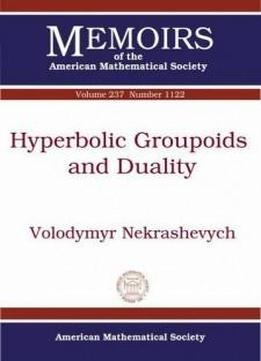# Hyperbolic Groupoids And Duality (memoirs Of The American Mathematical Society) by Volodymyr Nekrashevych / 2015 / English / PDF

The author introduces a notion of hyperbolic groupoids, generalizing the notion of a Gromov hyperbolic group. Examples of hyperbolic groupoids include actions of Gromov hyperbolic groups on their boundaries, pseudogroups generated by expanding self-coverings, natural pseudogroups acting on leaves of stable (or unstable) foliation of an Anosov diffeomorphism, etc. The author describes a duality theory for hyperbolic groupoids. He shows that for every hyperbolic groupoid \$\mathfrak{G}\$ there is a naturally defined dual groupoid \$\mathfrak{G}^\top\$ acting on the Gromov boundary of a Cayley graph of \$\mathfrak{G}\$. The groupoid \$\mathfrak{G}^\top\$ is also hyperbolic and such that \$(\mathfrak{G}^\top)^\top\$ is equivalent to \$\mathfrak{G}\$. Several classes of examples of hyperbolic groupoids and their applications are discussed.

The author introduces a notion of hyperbolic groupoids, generalizing the notion of a Gromov hyperbolic group. Examples of hyperbolic groupoids include actions of Gromov hyperbolic groups on their boundaries, pseudogroups generated by expanding self-coverings, natural pseudogroups acting on leaves of stable (or unstable) foliation of an Anosov diffeomorphism, etc. The author describes a duality theory for hyperbolic groupoids. He shows that for every hyperbolic groupoid \$\mathfrak{G}\$ there is a naturally defined dual groupoid \$\mathfrak{G}^\top\$ acting on the Gromov boundary of a Cayley graph of \$\mathfrak{G}\$. The groupoid \$\mathfrak{G}^\top\$ is also hyperbolic and such that \$(\mathfrak{G}^\top)^\top\$ is equivalent to \$\mathfrak{G}\$. Several classes of examples of hyperbolic groupoids and their applications are discussed.

views: 619